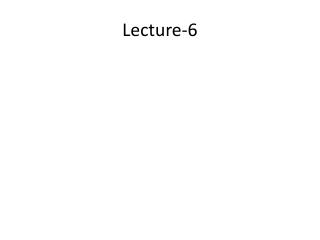Download PresentationLecture-6

# Lecture-6

Download Presentation## Lecture-6

- - - - - - - - - - - - - - - - - - - - - - - - - - - E N D - - - - - - - - - - - - - - - - - - - - - - - - - - -
##### Presentation Transcript

1. Lecture-6

2. Profit Maximization and Cost Minimization • The main objective of firm is to maximize profit. • Profit is the gap or difference between revenue and cost. That is Profit= Revenue ( R) - Cost (C) So the firm’s objective is to make this gap as large as possible which will ensure profit maximization. First lets find out how we can find the revenue of a firm. If the output/production of firm is expressed as Q and price of per unit of the output is expressed as P then revenue will be R= PQ That is revenue is price times quantity. We already know that production function can be written as Q = f (K,L) where we assume that capital and labor are the two inputs. So we can write R= P f(K,L) If total cost is C= w. L + r.K ( Where w is the wage and r is the interest rate) Then the profit becomes Profit= P f(K,L)- (w. L + r.K) Here P , w and r are constant. So firm can only choose the amount of L and K. The firm will choose labor and capital in such a way that the gap between total revenue and total cost is as large as possible that means profit is maximized.

3. Marginal cost • Marginal cost (MC): Marginal cost is the increment, or addition, to cost that results from producing one more unit of output

4. Marginal Revenue • Marginal Revenue (MR) :Marginal revenue is the increment, or addition, to revenue that results from producing one more unit of output

5. ‘Rule of Profit Maximization’ When a producer is maximizing profit, there is no incentive for him to deviate that means increase or decrease production. This implies that if a certain amount of production leads to profit maximization then the producer will not change the level of production. Producer’s decision about production at margin and its relation with profit maximization: Producer’s decision at margin means that how does a producer take decision about additional production( whether to increase or decrease or keep production same ). If we find a situation where the producer does not have any incentive to change production then we call this profit maximizing situation.

6. There can be three situation Case 1: MR > MC • If marginal revenue exceeds marginal cost, the production of an additional unit of output generate more revenue than cost from that additional unit. • In this case, a firm is expected to increase its level of production to increase its profits. Case 2: MR < MC • If marginal cost exceeds marginal revenue, the production of the last unit of output costs more than the additional revenue generated by that unit. • In this case, firms can increase their profits by producing less.

7. (Golden Rule of Profit maximization) A profit-maximizing firm will produce more output when MR > MC and less output when MR < MC. Case 3: MR = MC • If MR = MC, however, the firm has no incentive to produce either more or less output. • The firm's profits are maximized at the level of output at which MR = MC. This is know as golden rule of profit maximization.